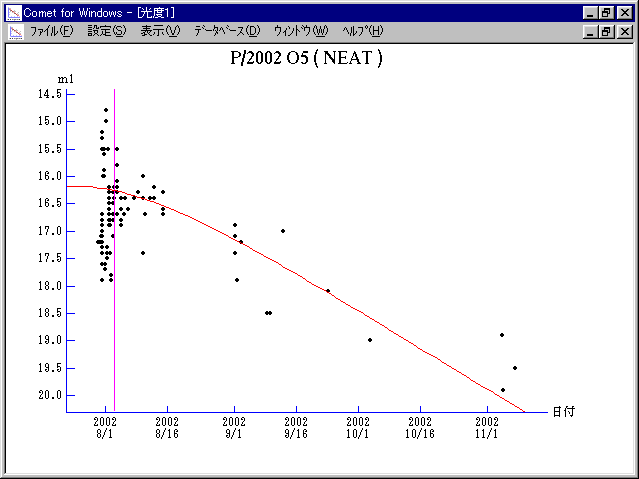# \$B%K!<%HWB@1(B

189P/NEAT (2002)###\$B%W%m%U%#!<%k(B

 \$BId9f(B 189P/2002 O5 \$BH/8+F|(B 2002\$BG/(B7\$B7n(B30\$BF|(B \$BH/8+8wEY(B 17.2\$BEy(B \$BH/8+ Near-Earth-Asteroid Tracking Team (Jet Propulsion Laboratory)

###\$B50F;MWAG(B

```                    Epoch = 2002 July 25.0 TT
T = 2002 Aug.  3.10144 TT        Peri. =  15.30571
e = 0.5973957                    Node  = 282.20995 2000.0
q = 1.1742394 AU                 Incl. =  20.39966
a =  2.9166089 AU   n = 0.19787311   P =   4.98 years
```

###\$B@1?^(B###\$B8wEYJQ2=(B

```        m1 = 18.0 + 5 log\$B&\$(B + 10.0 log r
```##### \$B50F;MWAG\$O!"(B IAUC 8856 \$B\$K7G:\\$5\$l\$?\$b\$N\$G\$9!#(B \$B@1?^\$O(B StellaNavigator Ver.2.0 for Windows (\$B%"%9%H%m%"!<%D(B \$BJTCx(B / \$B%"%9%-!<=PHG6I4)(B) \$B\$G:n@.\$7\$?\$b\$N\$G\$9!#(B \$B8wEY%0%i%U\$O(BComet for Windows\$B\$G:n@.\$7\$?\$b\$N\$G\$9!#(B